Courses

# Test: Shear Force & Bending Moment - 3

## 10 Questions MCQ Test Topicwise Question Bank for Civil Engineering | Test: Shear Force & Bending Moment - 3

Description
This mock test of Test: Shear Force & Bending Moment - 3 for Civil Engineering (CE) helps you for every Civil Engineering (CE) entrance exam. This contains 10 Multiple Choice Questions for Civil Engineering (CE) Test: Shear Force & Bending Moment - 3 (mcq) to study with solutions a complete question bank. The solved questions answers in this Test: Shear Force & Bending Moment - 3 quiz give you a good mix of easy questions and tough questions. Civil Engineering (CE) students definitely take this Test: Shear Force & Bending Moment - 3 exercise for a better result in the exam. You can find other Test: Shear Force & Bending Moment - 3 extra questions, long questions & short questions for Civil Engineering (CE) on EduRev as well by searching above.
QUESTION: 1

Solution:
QUESTION: 2

Solution:
QUESTION: 3

### The correct shear force diagram for the beam shown in the figure is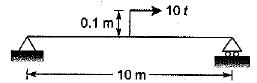Solution:
QUESTION: 4

A simply supported beam of span l is loaded (as shown) with a udl of intensity w1 per unit length at A and w2 per unit length at B the shear force at the support B is given by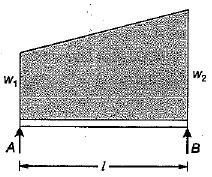Solution:
QUESTION: 5

The beam shown in the given figure has a design value of bending moment of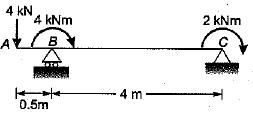Solution:
QUESTION: 6

A cantilever beam is subjected to moments as shown in the given figure. The BM diagram for the beam will be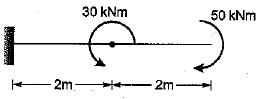Solution: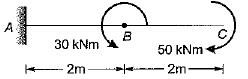The bending moment due to 50 kNm moment would be hogging. Similarly the bending moment due to 30 kNm would be sagging. Therefore the bending moment diagram is,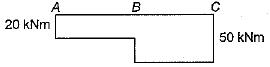QUESTION: 7

The fixed end moment MA of the beam shown in the given figure is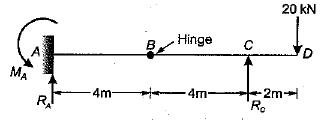Solution: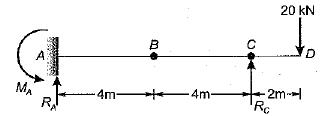∑FV = 0
⇒ RA + RC = 20
MB = 0 [from right]
∴ RC x 4 - 20 x 6 = 0
⇒ Rc x 4 = 20 x 6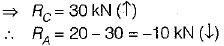MB = 0 [from left]
RA x 4 - MA = 0
⇒ MA = RA x 4 = -10 x 4
= - 40 kNm
The fixed end moment at A will be in clockwise direction, opposite to the direction shown in the figure.
∴ MA = -40 kNm

QUESTION: 8

A simply supported beam with equal overhang ori both sides is loaded as shown in the figure. If the bending moment at mid-span is zero, then the percentage overhang on each side will be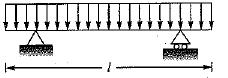Solution:

Let the overhang percentage in 100p.
So overhang = pl
The shear force diagram will be,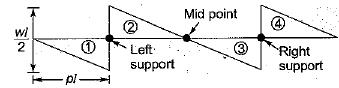For B.M. at mid point to be zero, area of shear force diagram under (1) should be equal to that of (2),
1/2w(pl)2 = 1/2w[(0.5 - P)l]2
or p2 = (0.5 - p)2 = 0.25 - p + p2
∴ p = 0.25 or 25%

QUESTION: 9

A propped cantilever beam shown in the figure given is having interna! hinge at its mid-span. Which one of the following is the shape of bending moment diagram for the given loading?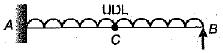Solution:

Due to hinge at C the part CB of beam be have s as simply supported beam. Thus reaction at B will be in upward direction and equals half of the load in span BC.
Span CA be haves as a cantilever beam. There fore B.M.D. will be sagging in BC and hogging in AC and it will be parabolic.
For a cantiiever B.M.D. will be as shown below.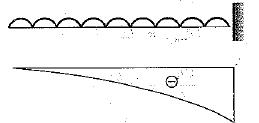Combining all the arguments answer will be (d) which is given with BM plotted on tension side.

QUESTION: 10

In the case of l-section, the web resists mainly

Solution:

In the case of (-section, nearly 80-90% shear force, is resisted by web and only 10-20% is resisted by flanges, whereas in bending. 80-90% moment is resisted by flanges and only 10-20% by the web.